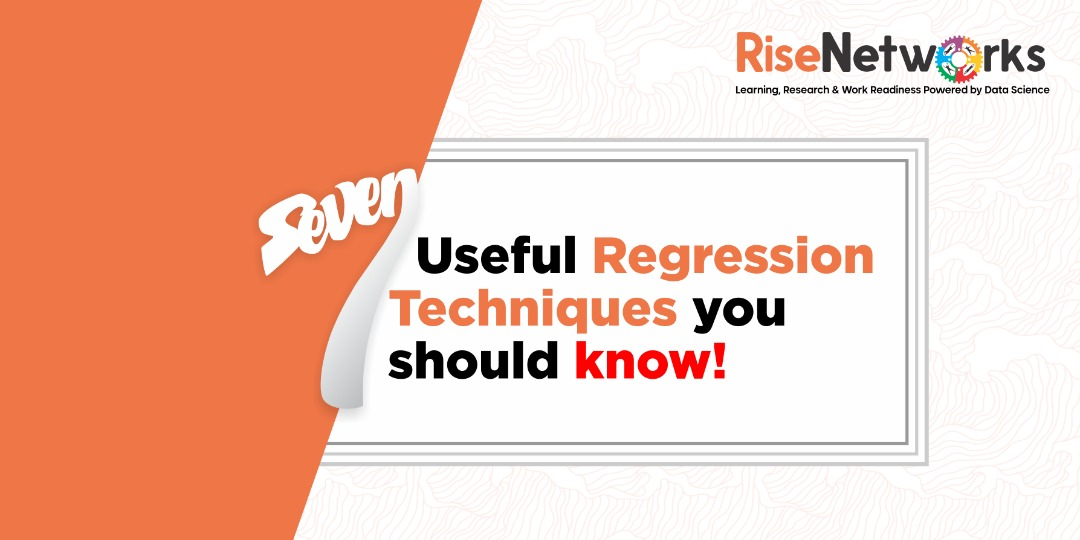Rise Networks# 7 Useful Regression Techniques you should know!

## Introduction

The first algorithms people learn in data science are usually linear and logistic regressions. Because of their widespread use, many analysts mistakenly believe that they are the only type of regression. Those who are slightly more interested believe that regression analysis is the most important of all types.

The truth is that there are countless types of regressions that can be carried out. Each form has its significance and a set of circumstances under which it should be used. In this article, I’ve outlined the seven most popular types of regression used in data science in a straightforward manner.

I also hope that by reading this post, individuals would gain a better understanding of the range of regressions available.

## Regression Analysis

Regression analysis is a type of predictive modelling technique that looks into the relationship between a dependent (target) and an independent (s) variable (predictor). Forecasting, time series modelling, and determining the causal effect link between variables are all done with this technique. The association between rash driving and the number of road accidents caused by a driver, for example, is best explored using regression.

Regression analysis is a crucial tool for data modelling and analysis. In this case, we place a curve/line to the data points in such a way that the discrepancies between the data points’ distances from the curve/line are reduced. In the following parts, I’ll go through this in greater detail.

## Types of Regression Analysis

To produce predictions, you can use a variety of regression methods. Three metrics are primarily used to guide these strategies (number of independent variables, type of dependent variables and shape of regression line). In the next sections, we’ll go through each one in-depth.

1. Linear Regression

It’s one of the most well-known modelling approaches. When learning predictive modelling, linear regression is frequently one of the first topics that individuals choose. The dependent variable in this technique is continuous, the independent variable(s) might be continuous or discrete, and the regression line is linear.

Linear regression uses a best-fit straight line to establish a link between a dependent variable (Y) and one or more independent variables (X) (also known as a regression line).

It’s written as Y=a+b*X + e, where an is the intercept, b is the line’s slope, and e is the error term. Based on the predictor variable, this equation can be used to predict the value of the target variable (s).

2. Logistic Regression

The probability of event=Success and event=Failure is calculated using logistic regression. When the dependent variable is binary (0/ 1, True/ False, Yes/ No), we should apply logistic regression. The value of Y here ranges from 0 to 1.

We need to find a link function that is best suited for a binomial distribution (dependent variable) because we are working with one. It’s also a logit function. Instead of minimizing the sum of squared errors, the parameters in the equation above are chosen to maximize the likelihood of observing the sample values (like in ordinary regression).

3. Polynomial Regression

Polynomial Regression is the third step in the regression process.

If the power of the independent variable is more than 1, the regression equation is a polynomial regression equation. A polynomial equation is represented by the equation below:

y=a+b*x^2

The best fit line in this regression procedure is not a straight line. Rather, it’s a curve that fits the data points.

4. Regression in Steps

When dealing with several independent variables, this type of regression is used. The selection of independent variables is done with the help of an automatic method that does not require human interaction in this technique.

To accomplish this feat, statistical parameters like as R-square, t-stats, and the AIC metric are used to identify significant factors. Stepwise regression is a method of fitting a regression model by adding or removing co-variates one at a time, according to a set of criteria. The following are some of the most commonly used Stepwise regression methods:

Stepwise regression, in its most basic form, accomplishes two goals. For each stage, it adds and removes predictors as needed.

Forward selection begins with the model’s most important predictor and adds a variable at each step.

5. Ridge Regression

Ridge Regression is a technique for dealing with multicollinear data (independent variables are highly correlated). Even though the least-squares estimates (OLS) are unbiased in multicollinearity, their variances are substantial, causing the observed value to diverge significantly from the true value. Ridge regression reduces standard errors by adding a degree of bias to the regression estimates.

6. Lasso Regression

Similar to Ridge Regression, Lasso (Least Absolute Shrinkage and Selection Operator) also penalizes the absolute size of the regression coefficients. In addition, it is capable of reducing the variability and improving the accuracy of linear regression models. Look at the equation below: Lasso regression differs from ridge regression in a way that it uses absolute values in the penalty function, instead of squares. This leads to penalizing (or equivalently constraining the sum of the absolute values of the estimates) values which causes some of the parameter estimates to turn out exactly zero. The larger the penalty applied, the further the estimates get shrunk towards absolute zero. This results in variable selection out of given n variables.

7. ElasticNet Regression

ElasticNet is a hybrid of Lasso and Ridge Regression techniques. It is trained with L1 and L2 prior as regularizers. Elastic-net is useful when there are multiple features that are correlated. Lasso is likely to pick one of these at random, while elastic-net is likely to pick both.

A practical advantage of trading-off between Lasso and Ridge is that, it allows Elastic-Net to inherit some of Ridge’s stability under rotation.